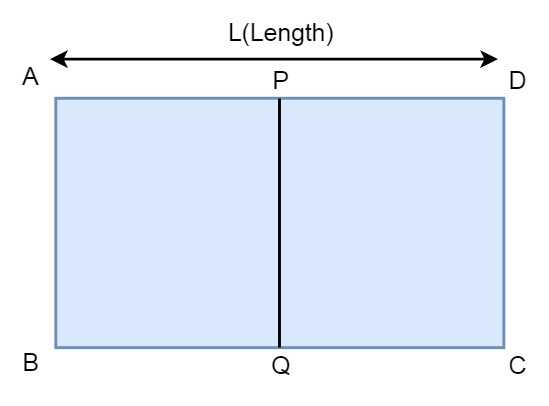# Find Corners of Rectangle using mid points in C++

C++Server Side ProgrammingProgramming

#### C in Depth: The Complete C Programming Guide for Beginners

45 Lectures 4.5 hours

#### Practical C++: Learn C++ Basics Step by Step

Most Popular

50 Lectures 4.5 hours

#### Master C and Embedded C Programming- Learn as you go

66 Lectures 5.5 hours

Suppose we have a rectangle ABCD, but we have only the coordinates of the mid points P and Q, and the length of the rectangle L.Our task is to find the coordinates of A, B, C and D using the coordinates of P and Q, and the length of side L. For example, if P is (1, 0), and Q is (1, 2), and L is 2, then A, B, C, D will be respectively (0, 0), (0, 2), (2, 2). (2, 0).

There can be three cases that can occur.

• The rectangle is horizontal, so AD and BC are parallel to X axis
• The rectangle is vertical, so AD and BC are parallel to Y axis
• Rectangle is inclined at a certain angle with the axes.

For the third case, we have to find the slope using the coordinates of P and Q. If we get the slope of AD, then we can generate the straight line equation passes through AD, then using the distance formula we will get the result.

$$Slope of AD,m=\frac{px-qx}{py-qy}$$ $$Displacement\:along\:x\:axis, dx=\frac{L}{2\sqrt{1+m^{2}}}$$ $$Displacement\:along\:y\:axis, dy=\frac{mL}{2\sqrt{1+m^{2}}}$$

## Example

Live Demo

#include <iostream>
#include <cmath>
using namespace std;
class Point {
public:
float x, y;
Point(float a = 0.0f, float b = 0.0f) {
x = a, y = b;
}
};
void printCorners(Point p, Point q, float l) {
Point a, b, c, d;
if (p.x == q.x) {
a.x = p.x - (l/2.0);
d.x = p.x + (l/2.0);
a.y = d.y = p.y;
b.x = q.x - (l/2.0);
c.x = q.x + (l/2.0);
b.y = c.y = q.y;
}else if (p.y == q.y) {
a.y = p.y - (l/2.0);
d.y = p.y + (l/2.0);
a.x = d.x = p.x;
b.y = q.y - (l/2.0);
c.y = q.y + (l/2.0);
b.x = c.x = q.x;
}else{
float m = (p.x-q.x)/float(q.y-p.y);
float dx = (l /sqrt(1+(m*m))) *0.5 ;
float dy = m*dx;
a.x = p.x - dx;
a.y = p.y - dy;
d.x = p.x + dx;
d.y = p.y + dy;
b.x = q.x - dx;
b.y = q.y - dy;
c.x = q.x + dx;
c.y = q.y + dy;
}
cout << "A (" << a.x << ", " << a.y << ")\n"
<< "B (" << b.x << ", " << b.y << ")\n"
<< "C (" << c.x << ", " << c.y << ")\n"
<< "D (" << d.x << ", " << d.y << ")\n";
}
int main() {
Point p(1, 1), q(-1, -1);
printCorners(p, q, 2*sqrt(2));
}

## Output

A (0, 2)
B (-2, 0)
C (0, -2)
D (2, 0)